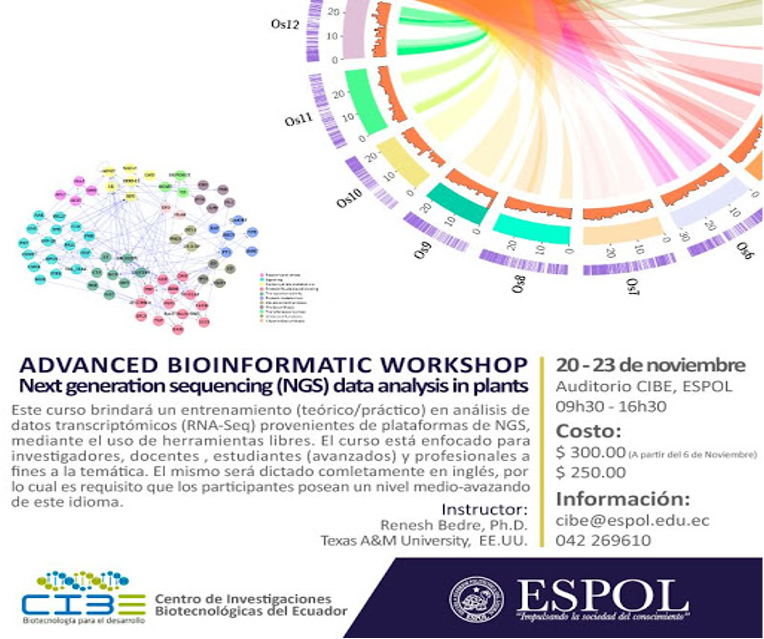## Create a gene counts matrix from featureCounts

Generate a gene counts matrix when featureCounts run separately on individual aligned files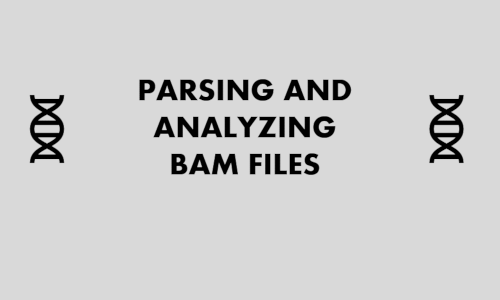## Parsing and analyzing BAM files

SAMtools for manipulation of BAM files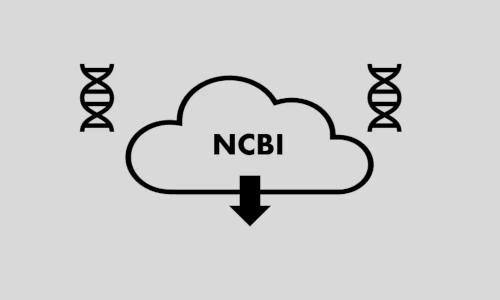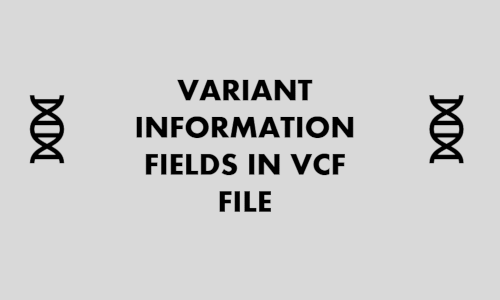## A guide to understanding the variant information fields in variant call format (VCF) file

VCF fields information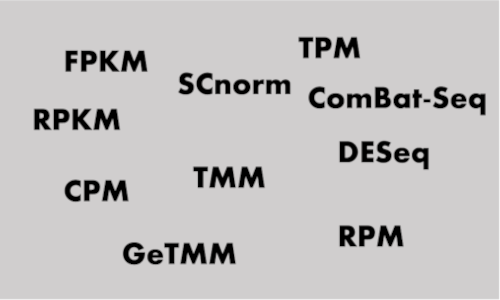## Gene expression units explained: RPM, RPKM, FPKM, TPM, DESeq, TMM, SCnorm, GeTMM, and ComBat-Seq

bulk and single-cell RNA-seq expression units, count normalization, formula, examples in Python, gene quantification, batch effects, and between-sample and w...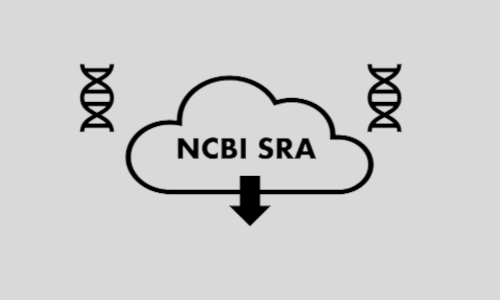## How to use NCBI SRA Toolkit effectively?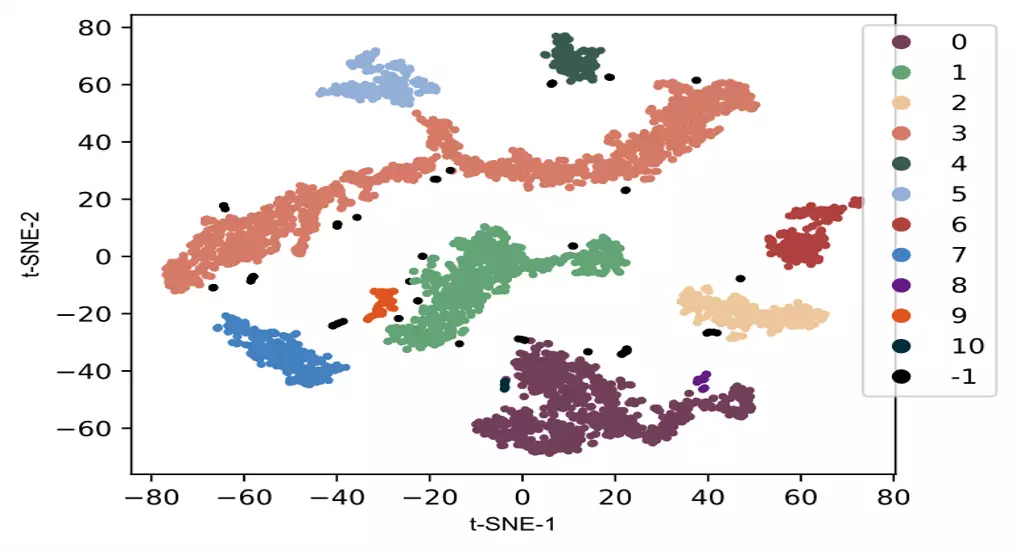## t-SNE in Python [single cell RNA-seq example and hyperparameter optimization]

t-SNE using sklearn package. This article explains the basics of t-SNE, differences between t-SNE and PCA, example using scRNA-seq data, and results interpre...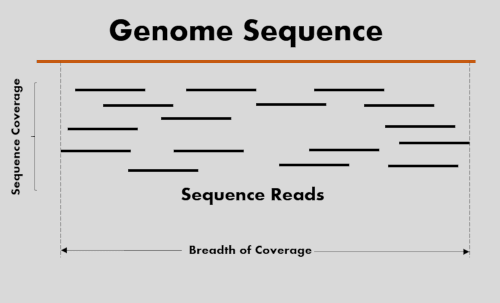## Sequencing coverage and breadth of coverage

High-through sequencing coverage calculation and coverage recommendations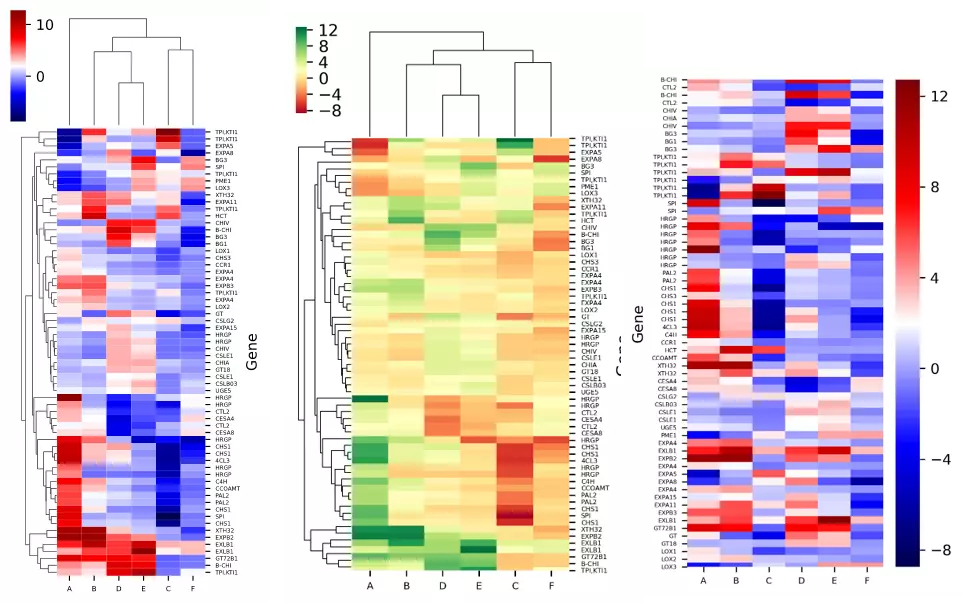## Heatmap in Python

Heatmap and hierarchical clustering visualization in Python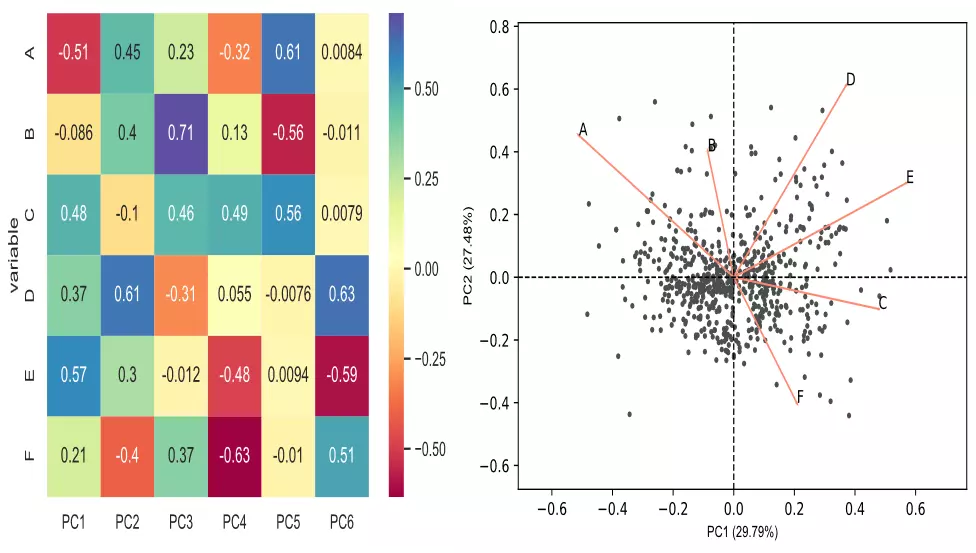## Performing and visualizing the Principal component analysis (PCA) from PCA function and scratch in Python

PCA using sklearn package. This article explains the basics of PCA, sample size requirement, data standardization, and interpretation of the PCA results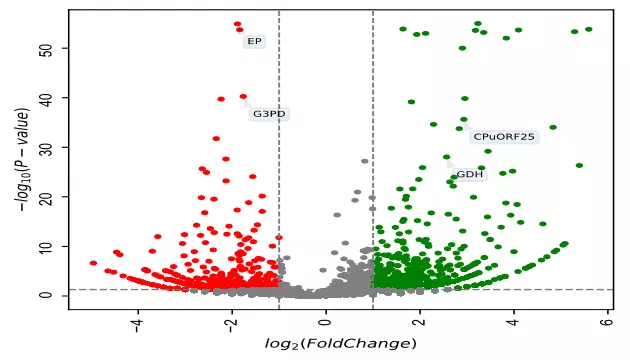## Volcano plot in Python

Volcano plot using bioinfokit package. This article explains the visualization of volcano plots for gene expression data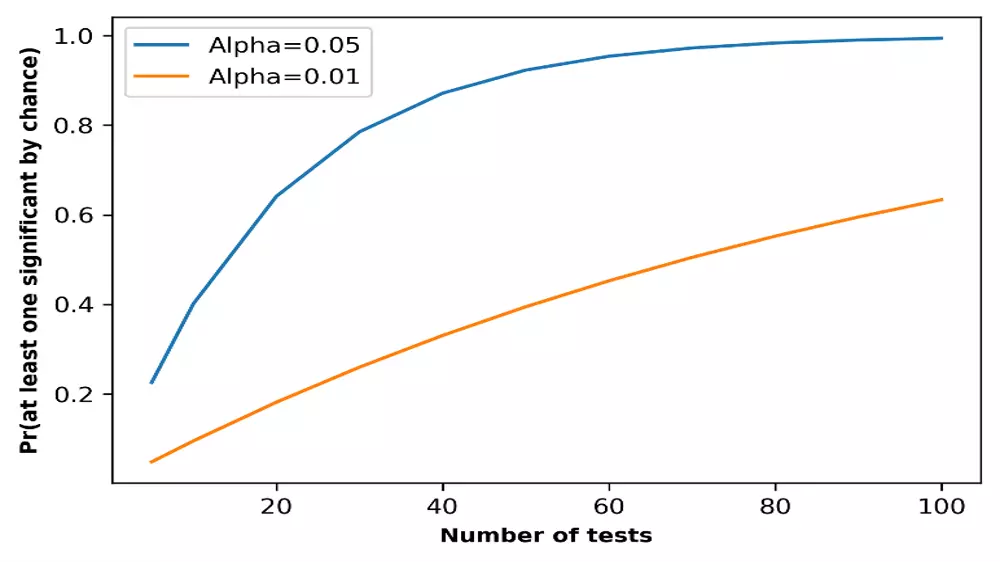## Multiple hypothesis testing problem in Bioinformatics

Multiple hypothesis testing and corrections, type I and II errors, false discovery rate, Bonferroni correction, and Benjamini/Hochberg correction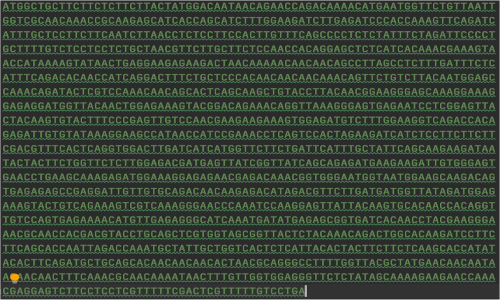## Bioinformatics file readers and processing (FASTA, FASTQ, and VCF)

Biological data handling and processing using Python codes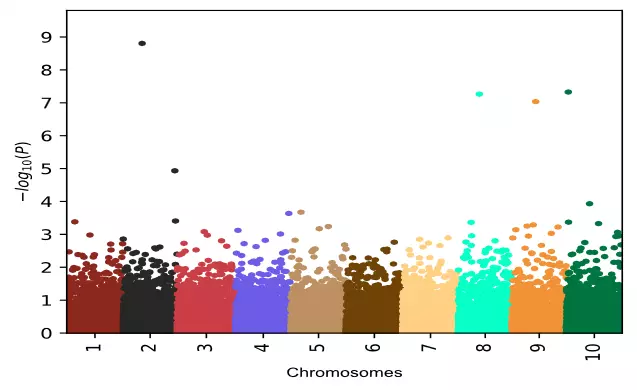## Manhattan plot

Introduction, analysis, and visualization of Manhattan plot in Python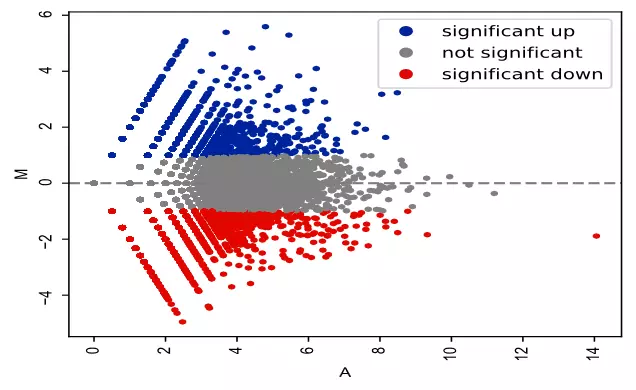## MA plot to visualize gene expression data using Python

MA plot basics, analysis, and visualization

## Split the sequence into smaller subsequences

Split the nucleotide sequence into smaller sequences with defined size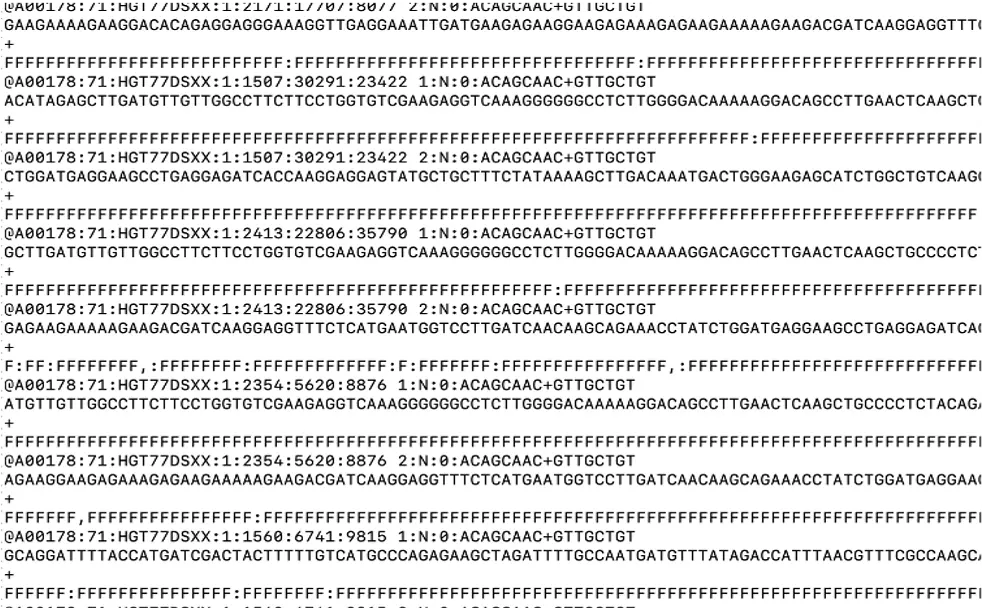## FASTQ Sequence Quality Format

FASTQ sequence example, quality formats, and quality format detection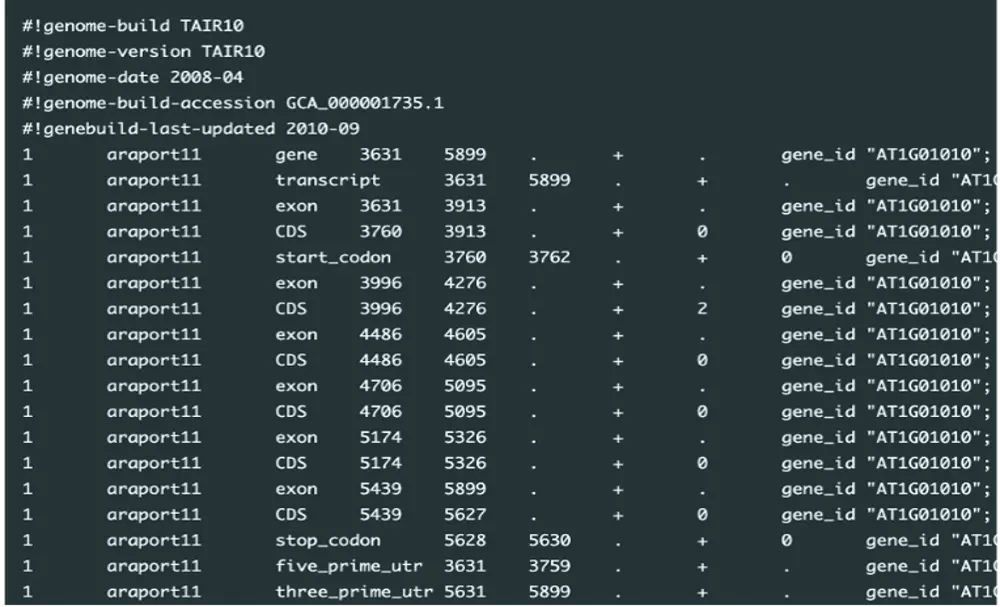## Convert GFF3 to GTF file format

Introduction to GFF3 and GTF files, and their interconversions using Python code## File format conversions

FASTQ to FASTA, GFF3 to GTF, HMM to CSV, TAB to CSV, and CSV to TAB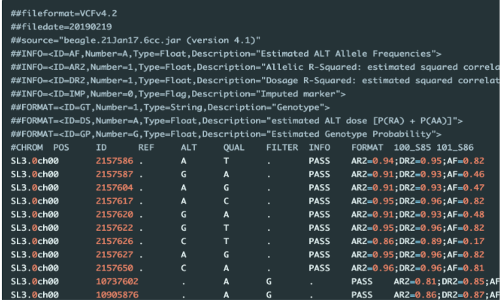## Annotation of genetic variants (SNPs/InDels) in VCF file

Genetic variant annotation for variant location in the genome, associated genes, and their gene functions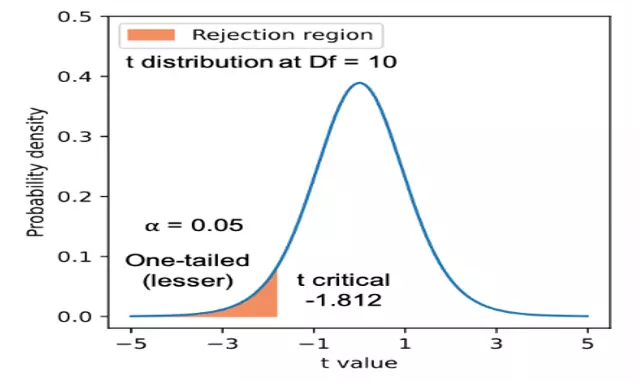## Statistical hypothesis testing, types of errors, and interpretation of p values

Learn how to propose null and alternate hypotheses, perform the statistical analysis, and interpret the results

## Query pandas DataFrame to select rows based on value and condition matching

Learn how to query pandas DataFrame to select rows based on exact match, partial match, and conditional match in pandas DataFrame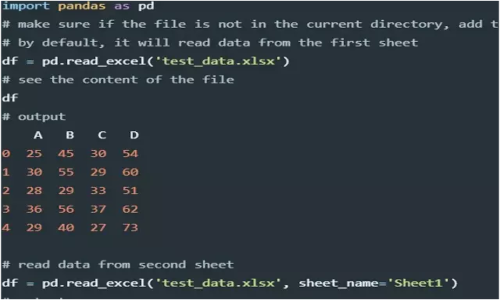## Import small and large data using pandas (CSV, Excel, Tab, JSON, SQL, and Parquet files)

learn how to import CSV, Excel, Tab, JSON, and SQL files in pandas for data analysis and visualization## Checking and handling missing values (NaN) in pandas

Analyse and handle null or missing values in pandas series and dataframe## Left, right, outer and inner joins on pandas dataframes

learn to join pandas dataframes in multiple ways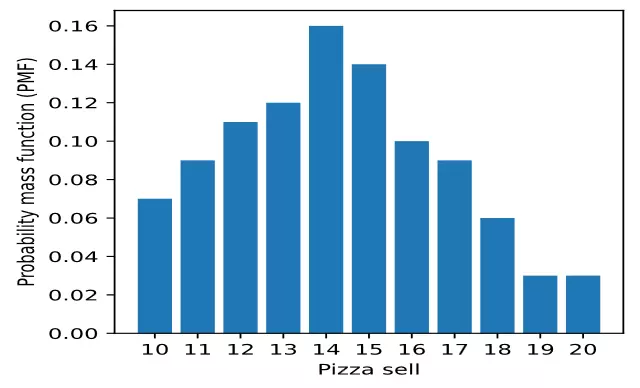## Find probabilities using discrete and continuous probability distributions

Learn how to use probability distributions, probability mass function (PMF), cumulative distribution function (CDF), and probability density function (PDF)

## 2020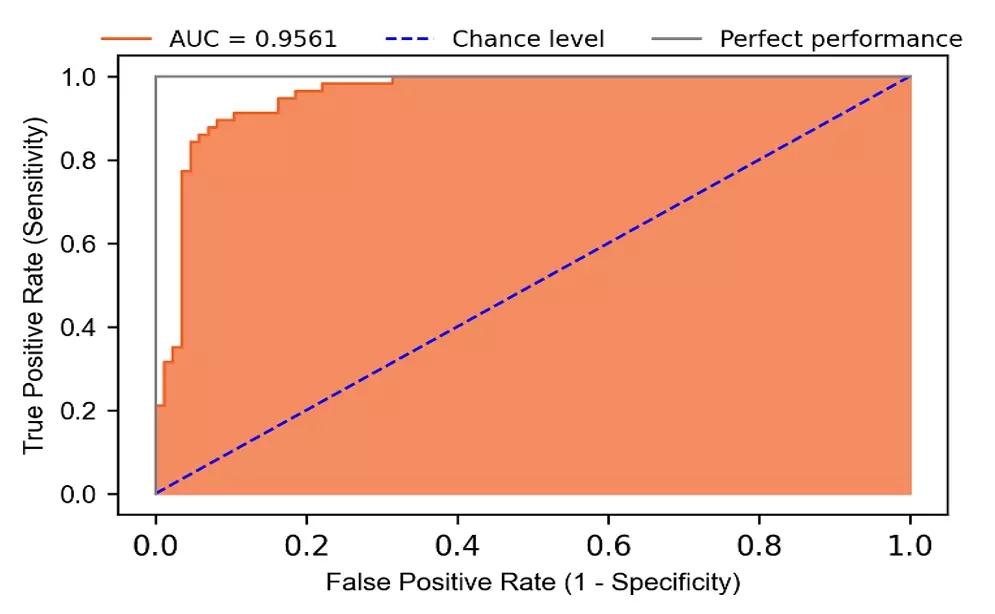## Logistic regression in Python (feature selection, model fitting, and prediction)

Logistic regression for prediction of breast cancer, assumptions, feature selection, model fitting, model accuracy, and interpretation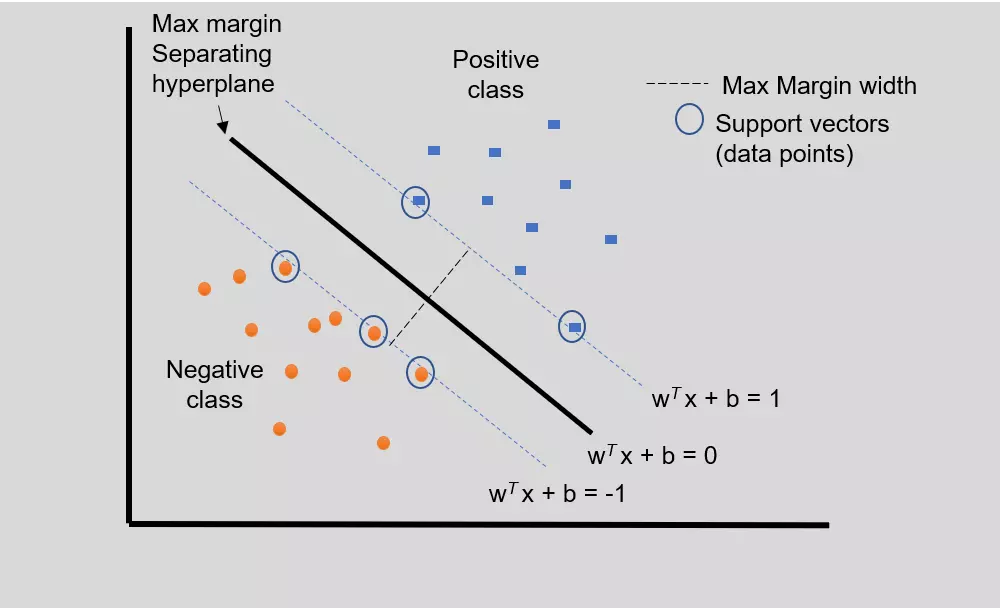## Support Vector Machine (SVM) basics and implementation in Python

Implementation of Support vector machine (SVM) in Python for prediction of heart disease. Learn SVM basics, model fitting, model accuracy, and interpretation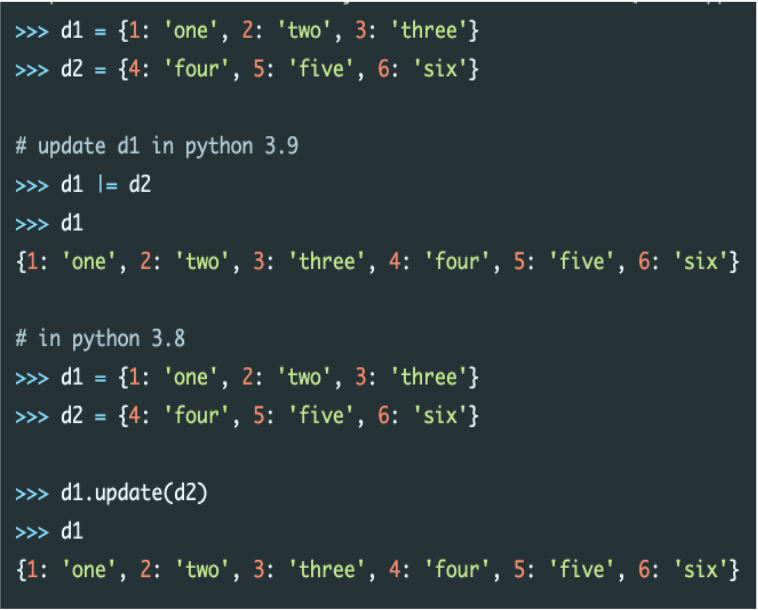## New good features in Python 3.9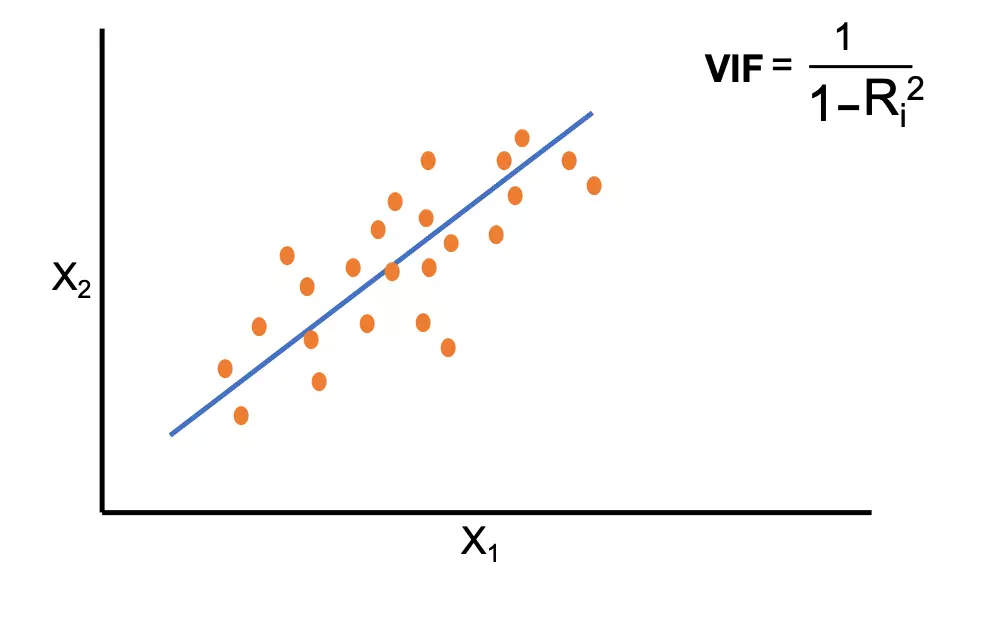## Multicollinearity and variance inflation factor (VIF) in the regression model

Multicollinearity refers to the significant correlation among the independent variables in the regression model. Variance Inflation Factor (VIF) helps to dia...## Multiple linear regression (MLR)

Multiple regression analysis using statsmodels. Learn how to define regression model, assumptions, metrics evaluation, and interpretation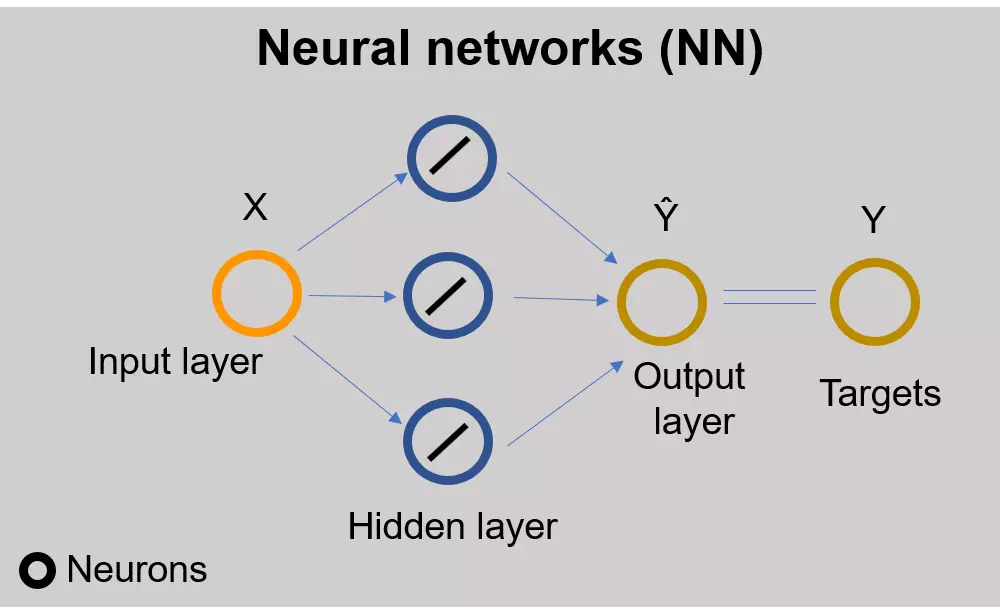## Linear Regression Using Neural Networks (PyTorch)

Linear regression using PyTorch## Linear regression basics and implementation in Python

Linear regression using statsmodels. Learn how to define, analyze and interpret the regression model.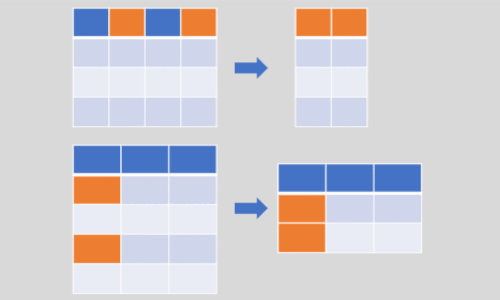## Multiple ways to select rows, columns, and subsets from pandas DataFrame

This article explains how to select rows, columns, and a subset of pandas DataFrame using various indexing operations and pandas functions

What is pandas?

## Pandas groupby function to group column values into list

Group dataframe rows into a list based on a common element from one column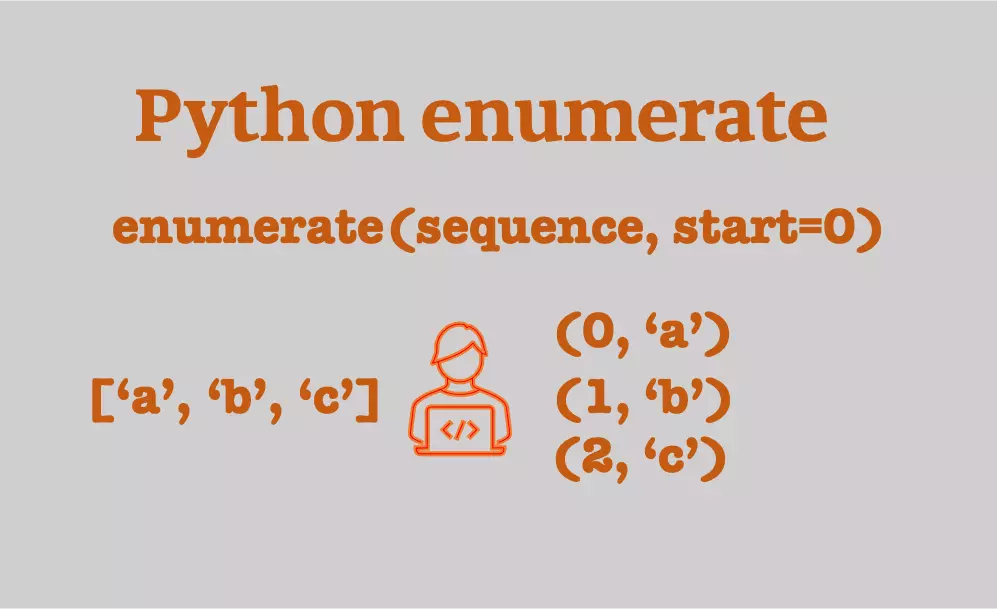## Python enumerate to simplify the looping

Python enumerate built-in function allows iterating over list and dictionary and helps to access its items along with index values## Python tuples

Python tuples initialization and operations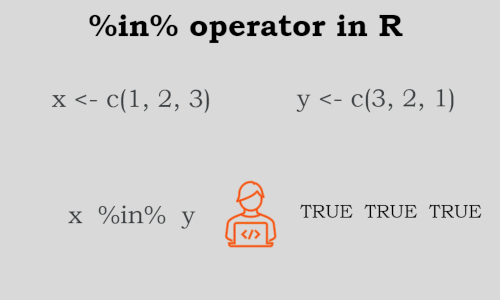## `%in%` and `%notin%` operators in R

Learn how to use %in% operator in R

## 8 different ways to get column names from pandas DataFrame

Learn different methods to get column names from pandas DataFrame

## 5 different ways to rename column names in pandas DataFrame

Learn different methods to rename column names in pandas DataFrame

## 3 ways to get the last characters of a string in Python

Learn how to get last any number of characters of the strings in Python

## Query pandas DataFrame to split the values in column

Learn how to split the value in pandas DataFrame column and create new columns

## 3 easy ways to remove the last characters of a string in Python

Learn how to get remove any number of characters of the strings in Python

## Chi-square (χ2) test in Python (Pearson Chi-square test)

Pearson Chi-square test, chi-square goodness of fit test, formula, assumptions, example in Python, and interpretation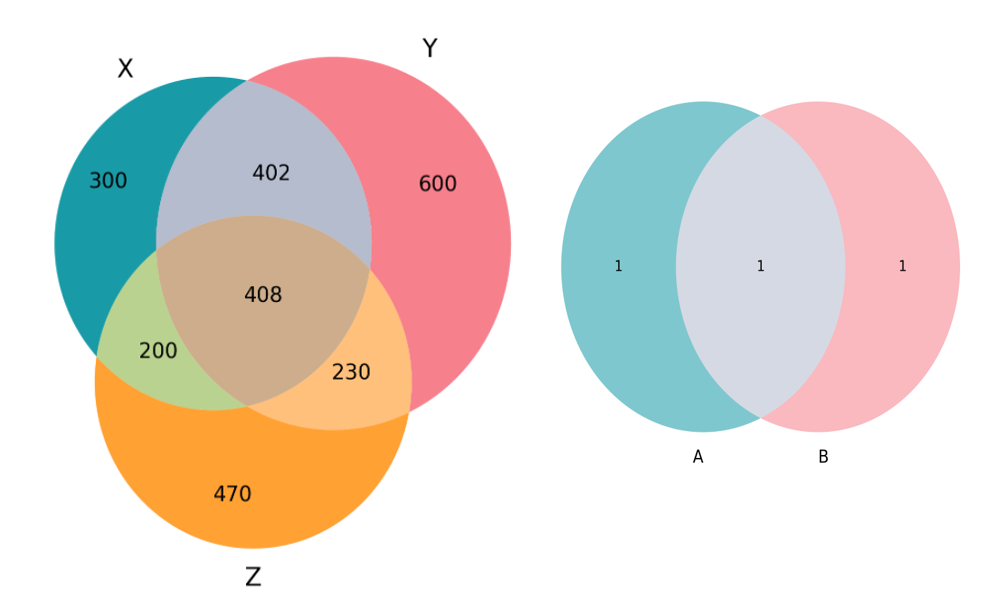## Venn diagrams in Python and R

Create two and three-way Venn diagrams in Python and R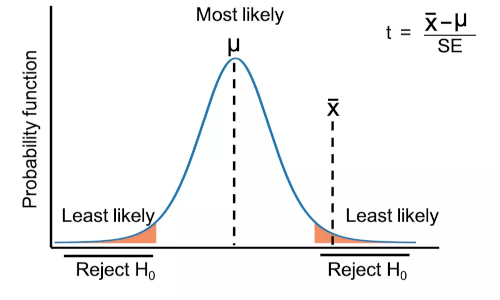## Perform three types of t-test in Python

Learn when to use t-test, types of t-test, assumptions, hypothesis, and formula for each type of test, and t-test calculation in Python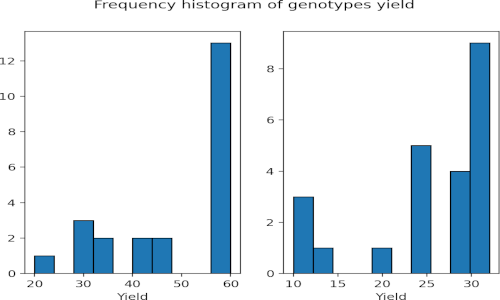## Mann-Whitney U test (Wilcoxon rank sum test) in Python

Learn when to use Mann-Whitney U test, assumptions, hypothesis, and formula, and test calculation in Python## Perform t-test from scratch in Python

Calculate three types of t-test from scratch## Reverse complementary

Reverse complementary of DNA sequences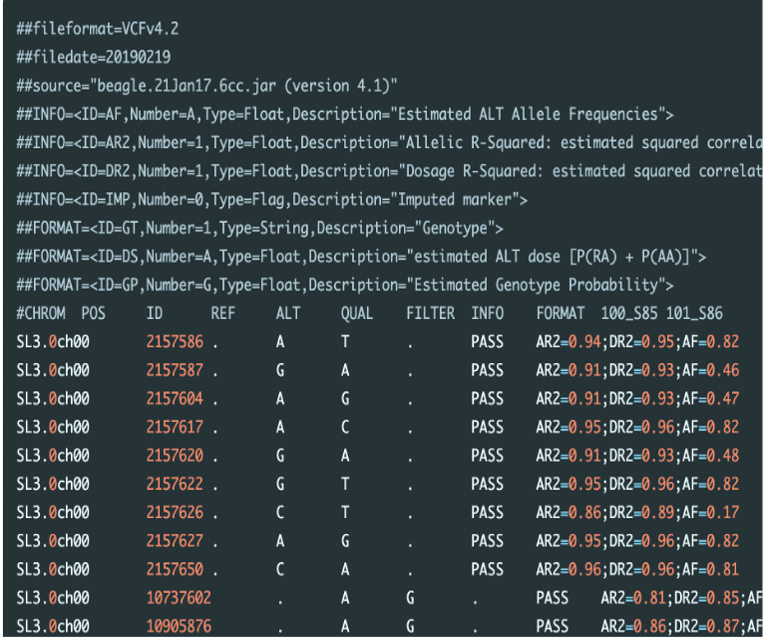## Concatenate and split VCF files

What is VCF file? VCF stands for variant call format It is a text file (file extension as .vcf) storing meta-information, marker and genotype data of ge...## Correlation analysis in Python

Correlation analysis using Python code

## 2018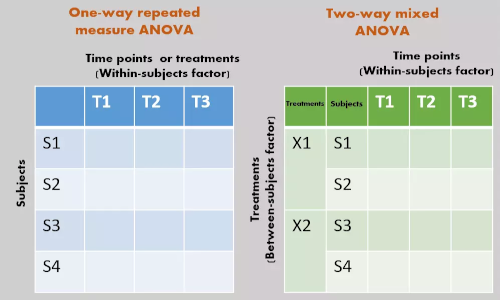## Repeated Measures ANOVA using Python and R (with examples)

Repeated Measure ANOVA in Python and R. This article explains repeated Measure ANOVA model, multiple pairwise comparisons, and results interpretation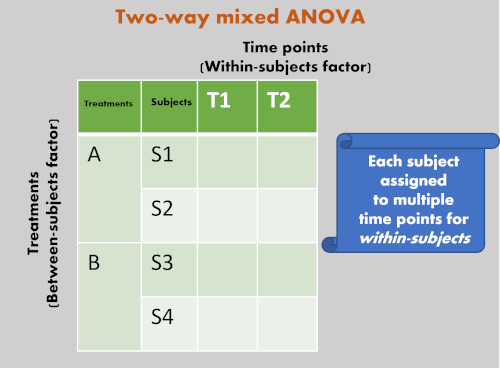## Mixed ANOVA using Python and R (with examples)

Learn to perform mixed ANOVA, check assumptions, and post-hoc tests for significant interactions and main effects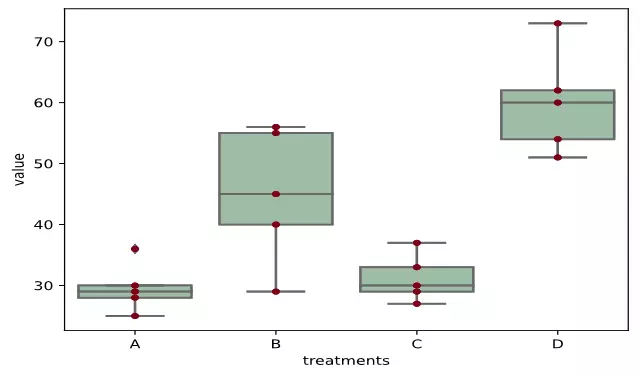## ANOVA using Python (with examples)

One and two-way ANOVA in Python. This article explains ANOVA model, formula, calculation, multiple pairwise comparisons, and results interpretation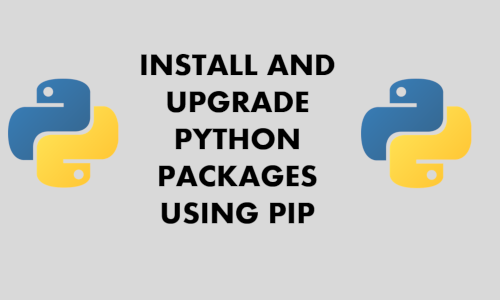## Install and upgrade Python packages using pip and virtual environment on Windows, Linux, and Mac

Learn how to install, upgrade, and check versions of Python packages

## 2017